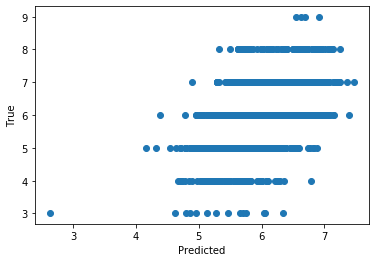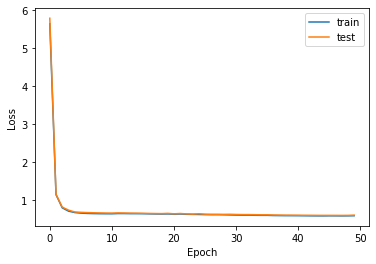# Exercise 4.1 - Solution¶

In :
import numpy as np
import matplotlib.pyplot as plt


Disclaimer

The book mistakently refers to the page for Exercise 4.2 when introducting Exercise 4.1, etc. Of course, these numbers should match: Book Exercise 4.1 is discussed under Exercise 4.1

Simple Network

We continue with the dataset first encountered in Exercise 3.2. Please refer to the discussion there for an introduction to the data and the learning objective.

Here, we manually implement a simple network architecture

In :
# The code snippet below is responsible for downloading the dataset
# - for example when running via Google Colab.
#
# with a local setup (in that case, ignore the !wget)

!wget https://archive.ics.uci.edu/ml/machine-learning-databases/wine-quality/winequality-white.csv

/bin/sh: wget: command not found

In :
# Before working with the data,

# load all examples from the file

print("data:", data.shape)

# Prepare for proper training
np.random.shuffle(data) # randomly sort examples

# take the first 3000 examples for training
# (remember array slicing from last week)
X_train = data[:3000,:11] # all features except last column
y_train = data[:3000,11]  # quality column

# and the remaining examples for testing
X_test = data[3000:,:11] # all features except last column
y_test = data[3000:,11] # quality column

print("First example:")
print("Features:", X_train)
print("Quality:", y_train)

data: (4898, 12)
First example:
Features: [6.300e+00 2.700e-01 1.800e-01 7.700e+00 4.800e-02 4.500e+01 1.860e+02
9.962e-01 3.230e+00 4.700e-01 9.000e+00]
Quality: 5.0


# Solutions¶

The goal is to implement the training of a neural network with one input layer, one hidden layer, and one output layer using gradient descent. We first (below) define the matrices and initialise with random values. We need W, b, W' and b'. The shapes will be:

• W: (number of hidden nodes, number of inputs) named W
• b: (number of hidden nodes) named b
• W': (number of hidden nodes) named Wp
• b': (one) named bp

• Implement a forward pass of the network as dnn (see below)
• Implement a function that uses one data point to update the weights using gradient descent. You can follow the update_weights skeleton below
• Now you can use the code below (training loop and evaluation) to train the network for multiple data points and even over several epochs. Try to find a set of hyperparameters (number of nodes in the hidden layer, learning rate, number of training epochs) that gives stable results. What is the best result (as measured by the loss on the training sample) you can get?
In :
# Initialise weights with suitable random distributions
hidden_nodes = 50 # number of nodes in the hidden layer
n_inputs = 11 # input features in the dataset

# how to initialise network parameters
W = np.random.randn(hidden_nodes,11)*np.sqrt(2./n_inputs)
b = np.random.randn(hidden_nodes)*np.sqrt(2./n_inputs)
Wp = np.random.randn(hidden_nodes)*np.sqrt(2./hidden_nodes)
bp = np.random.randn((1))

print(W.shape)

(50, 11)

In :
# You can use this implementation of the ReLu activation function
def relu(x):
return np.maximum(x, 0)

In :
def dnn(x,W,b,Wp,bp):
# SOLUTION
# sum_i W'_ki*Relu(sum_j W_ij*x_j + b_i) + b'_k
return np.dot(Wp, relu(np.dot(W,x) + b)) + bp

In :
def update_weights(x,y, W, b, Wp, bp):

lr = 0.00005

# SOLUTION

# Calculate the network output
phi = dnn(x,W,b,Wp,bp)

# Use the formulas derived to calculate the gradient for each of W,b,Wp,bp
delta_bp = 2 * (phi - y)
delta_Wp = 2 * (phi - y) * relu(np.dot(W,x) + b)
delta_b  = 2 * (phi - y) * Wp * np.heaviside(np.dot(W,x) + b, 0.5)
delta_W  = 2 * (phi - y) * np.outer(Wp * np.heaviside(np.dot(W,x) + b, 0.5), x)

# Update the weights/bias following the rule:  X_new = X_old - learning_rate * gradient
bp -= lr * delta_bp
Wp -= lr * delta_Wp
b  -= lr * delta_b
W  -= lr * delta_W

return -1 # no return value needed, you can modify the weights in-place


# Training loop and evaluation below¶

In :
# The code below implements the training.
# If you correctly implement  dnn and update_weights above,
# you should not need to change anything below.
# (apart from increasing the number of epochs)

train_losses = []
test_losses = []

# How many epochs to train
n_epochs = 50

# Loop over the epochs
for ep in range(n_epochs):

# Each epoch is a complete over the training data
for i in range(X_train.shape):

# pick one example
x = X_train[i]
y = y_train[i]

# use it to update the weights
update_weights(x,y,W,b,Wp,bp)

# Calculate predictions for the full training and testing sample
y_pred_train = [dnn(x,W,b,Wp,bp) for x in X_train]
y_pred = [dnn(x,W,b,Wp,bp) for x in X_test]

# Calculate aver loss / example over the epoch
train_loss = sum((y_pred_train-y_train)**2) / y_train.shape
test_loss = sum((y_pred-y_test)**2) / y_test.shape

# print some information
print("Epoch:",ep, "Train Loss:", train_loss, "Test Loss:", test_loss)

# and store the losses for later use
train_losses.append(train_loss)
test_losses.append(test_loss)

# After the training:

# Prepare scatter plot
y_pred = [dnn(x,W,b,Wp,bp) for x in X_test]

print("Best loss:", min(test_losses), "Final loss:", test_losses[-1])

print("Correlation coefficient:", np.corrcoef(y_pred,y_test)[0,1])
plt.scatter(y_pred_train,y_train)
plt.xlabel("Predicted")
plt.ylabel("True")
plt.show()

# Prepare and loss over time
plt.plot(train_losses,label="train")
plt.plot(test_losses,label="test")
plt.legend()
plt.xlabel("Epoch")
plt.ylabel("Loss")
plt.show()

Epoch: 0 Train Loss: 5.645403582072304 Test Loss: 5.789360719845873
Epoch: 1 Train Loss: 1.1533433753334428 Test Loss: 1.1637637243130456
Epoch: 2 Train Loss: 0.8064459925389651 Test Loss: 0.8256775797887149
Epoch: 3 Train Loss: 0.7194986944212736 Test Loss: 0.7463420451468886
Epoch: 4 Train Loss: 0.6823412559002283 Test Loss: 0.6977324105881756
Epoch: 5 Train Loss: 0.6664846138051684 Test Loss: 0.6876245778967164
Epoch: 6 Train Loss: 0.6587998893746104 Test Loss: 0.6813185867359125
Epoch: 7 Train Loss: 0.6542220249941378 Test Loss: 0.6763715413742601
Epoch: 8 Train Loss: 0.6515229736431492 Test Loss: 0.6726972058370031
Epoch: 9 Train Loss: 0.6500603789067503 Test Loss: 0.6696083273661432
Epoch: 10 Train Loss: 0.6493454499784376 Test Loss: 0.6681520553512201
Epoch: 11 Train Loss: 0.6555439871036638 Test Loss: 0.6725116282689468
Epoch: 12 Train Loss: 0.6550890293925202 Test Loss: 0.6699690448726485
Epoch: 13 Train Loss: 0.6526835138767458 Test Loss: 0.6670276424175429
Epoch: 14 Train Loss: 0.6524323996393876 Test Loss: 0.6657859446027024
Epoch: 15 Train Loss: 0.6508383266412704 Test Loss: 0.6634289610832423
Epoch: 16 Train Loss: 0.6475738523938723 Test Loss: 0.6588708333747422
Epoch: 17 Train Loss: 0.6448067370447748 Test Loss: 0.656277910633608
Epoch: 18 Train Loss: 0.6429866949447117 Test Loss: 0.6541361924738887
Epoch: 19 Train Loss: 0.6452836868194535 Test Loss: 0.6603206884328453
Epoch: 20 Train Loss: 0.6390966116784169 Test Loss: 0.6484080376897273
Epoch: 21 Train Loss: 0.643608872593549 Test Loss: 0.6568905242756689
Epoch: 22 Train Loss: 0.6365683454156014 Test Loss: 0.6459070379047909
Epoch: 23 Train Loss: 0.6344200247151789 Test Loss: 0.6405648728904523
Epoch: 24 Train Loss: 0.6307892457380325 Test Loss: 0.6502294953157811
Epoch: 25 Train Loss: 0.6271675389745466 Test Loss: 0.6394346181103574
Epoch: 26 Train Loss: 0.625209796186921 Test Loss: 0.6367168482951644
Epoch: 27 Train Loss: 0.6254154882759166 Test Loss: 0.6365726656263813
Epoch: 28 Train Loss: 0.6233292217982007 Test Loss: 0.6342570747926862
Epoch: 29 Train Loss: 0.6194417930925457 Test Loss: 0.6365037775275856
Epoch: 30 Train Loss: 0.6153231814680605 Test Loss: 0.6317002972628465
Epoch: 31 Train Loss: 0.6126120664039123 Test Loss: 0.6287678460188807
Epoch: 32 Train Loss: 0.6129166430079448 Test Loss: 0.6284321403254162
Epoch: 33 Train Loss: 0.6104762499877989 Test Loss: 0.6257073921750971
Epoch: 34 Train Loss: 0.6082635861912785 Test Loss: 0.6230077493420016
Epoch: 35 Train Loss: 0.6080842723192383 Test Loss: 0.6227811633866328
Epoch: 36 Train Loss: 0.6035597556499765 Test Loss: 0.6178078657920026
Epoch: 37 Train Loss: 0.6016812959797005 Test Loss: 0.6154486388653907
Epoch: 38 Train Loss: 0.6000701547553112 Test Loss: 0.6136773857670896
Epoch: 39 Train Loss: 0.5996881694114135 Test Loss: 0.6129554259443293
Epoch: 40 Train Loss: 0.59857725747447 Test Loss: 0.6122699599476488
Epoch: 41 Train Loss: 0.5970114764108893 Test Loss: 0.6105162649916903
Epoch: 42 Train Loss: 0.5952629839015641 Test Loss: 0.6094251196258232
Epoch: 43 Train Loss: 0.5940349734884387 Test Loss: 0.608027638521175
Epoch: 44 Train Loss: 0.5934948491666505 Test Loss: 0.6075563960754147
Epoch: 45 Train Loss: 0.5953789625146336 Test Loss: 0.6078046455326416
Epoch: 46 Train Loss: 0.5948276458373926 Test Loss: 0.607165242559313
Epoch: 47 Train Loss: 0.5934935232783387 Test Loss: 0.6065483328684542
Epoch: 48 Train Loss: 0.59473349370651 Test Loss: 0.6076145117172599
Epoch: 49 Train Loss: 0.5978180426888854 Test Loss: 0.6139122064546286
Best loss: 0.6065483328684542 Final loss: 0.6139122064546286
Correlation coefficient: 0.47560172145940866# Hint 1¶

We want a network with one hidden layer. As activiation in the hidden layer $\sigma$ we apply element-wise ReLu, while no activation is used for the output layer. The forward pass of the network then reads: $$\hat{y}=\mathbf{W}^{\prime} \sigma(\mathbf{W} \vec{x}+\vec{b})+b^{\prime}$$

# Hint 2¶

For the regression problem the objective function is the mean squared error between the prediction and the true label $y$: $$L=(\hat{y}-y)^{2}$$

Taking the partial derivatives - and diligently the applying chain rule - with respect to the different objects yields: \begin{aligned} \frac{\partial L}{\partial b^{\prime}} &=2(\hat{y}-y) \\ \frac{\partial L}{\partial b_{k}} &=2(\hat{y}-y) \mathbf{W}_{k}^{\prime} \theta\left(\sum_{i} \mathbf{W}_{i k} x_{i}+b_{k}\right) \\ \frac{\partial L}{\partial \mathbf{W}_{k}^{\prime}} &=2(\hat{y}-y) \sigma\left(\sum_{i} \mathbf{W}_{i k} x_{i}+b_{k}\right) \\ \frac{\partial L}{\partial \mathbf{W}_{k m}} &=2(\hat{y}-y) \mathbf{W}_{m}^{\prime} \theta\left(\sum_{i} \mathbf{W}_{i k} x_{i}+b_{m}\right) x_{k} \end{aligned}

Here, $\Theta$ denotes the Heaviside step function.

In [ ]: